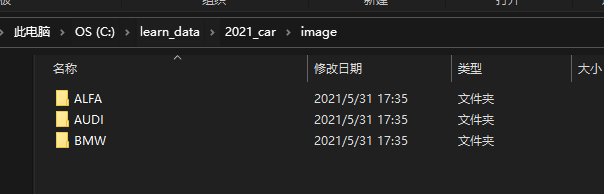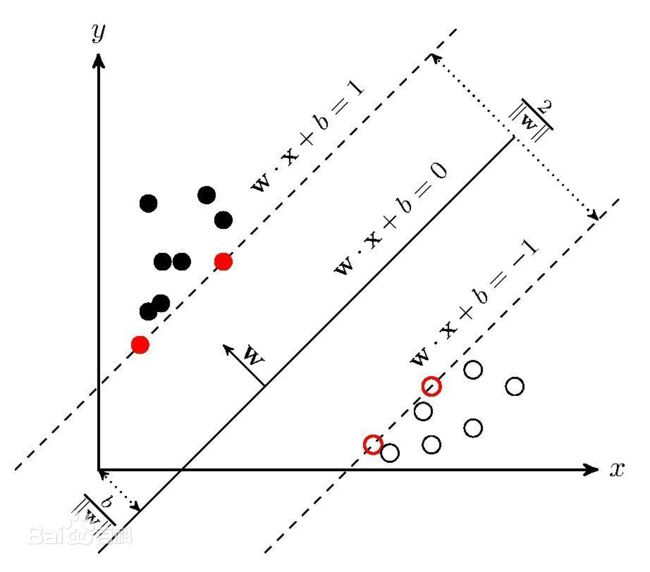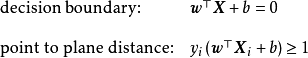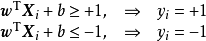• GridSearchCV用于python中的SVM
2020-12-18 19:56:06

我正在尝试学习如何为分类器找到最佳参数.所以,我使用GridSearchCV来解决多类分类问题.在Does not GridSearchCV support multi-class?上生成了一个虚拟代码我正在使用n_classes = 3的代码.

import numpy as np

from sklearn.datasets import make_classification

from sklearn.preprocessing import StandardScaler,label_binarize

from sklearn.svm import SVC

from sklearn.pipeline import make_pipeline

from sklearn.grid_search import GridSearchCV

from sklearn.metrics import accuracy_score, recall_score, f1_score, roc_auc_score, make_scorer

X, y = make_classification(n_samples=3000, n_features=10, weights=[0.1, 0.9, 0.3],n_classes=3, n_clusters_per_class=1,n_informative=2)

pipe = make_pipeline(StandardScaler(), SVC(kernel='rbf', class_weight='auto'))

param_space = dict(svc__C=np.logspace(-5,0,5), svc__gamma=np.logspace(-2, 2, 10))

f1_score

my_scorer = make_scorer(f1_score, greater_is_better=True)

gscv = GridSearchCV(pipe, param_space, scoring=my_scorer)

y = label_binarize(y, classes=[0, 1, 2])

for i in classes:

gscv.fit(X, y[i])

print gscv.best_params_

我正进入(状态：

ValueError: bad input shape (2000L, 3L)

我不知道为什么我会收到这个错误.我的目标是找到多类分类问题的最佳参数.

更多相关内容
• 下载后，安装好版本匹配的python3.6,numpy,scipy,matplot,sklearn,skimage等包，后直接可以运行，无需修改代码。运行后输入y，就可以实习自带的图像分类（小鸡，小鸭，蛇，猫等分类）。
• 主要介绍了Python实现基于SVM分类器的方法，文中通过示例代码介绍的非常详细，对大家的学习或者工作具有一定的参考学习价值，需要的朋友们下面随着小编来一起学习学习吧
• 对于分类，SVM最初用于解决二分类问题，分类问题可通过构建SVM分类器解决。SVM具有两大特点：1.寻求最优分类边界，即求解出能够正确划分训练数据集并且几何间隔最大的分离超平面，这是SVM的基本思想；2.基于核...
• ## svm图像分类python代码实现续

千次阅读 热门讨论 2021-05-31 17:41:32
svm图像分类python代码实现续 这篇博客诗接上前面一篇svm图像分类得一篇续集 svm分类代码如下 #os.system("pause") #Svm 训练： import sys import os import cv2 import numpy as np from sklearn.svm import ...

## svm图像分类python代码实现续

这篇博客诗接上前面一篇svm图像分类得一篇续集
svm分类代码如下


#os.system("pause")

#Svm 训练：
import sys
import os
import cv2
import numpy as np
from sklearn.svm import SVC
from sklearn.model_selection import train_test_split
import time
import pickle
#help(SVC)

SHAPE = (30, 30)
def getImageData(directory):
s = 1
feature_list = list()
label_list   = list()
num_classes = 0
for root, dirs, files in os.walk(directory):
for d in dirs:
num_classes += 1
images = os.listdir(root+d)
for image in images:
s += 1
label_list.append(d)
feature_list.append(extractFeaturesFromImage(root + d + "/" + image))

return np.asarray(feature_list), np.asarray(label_list)

def extractFeaturesFromImage(image_file):
img = cv2.resize(img, SHAPE, interpolation = cv2.INTER_CUBIC)
img = img.flatten()
img = img / np.mean(img)
return img

if __name__ == "__main__":

directory ="C:/learn_data/2021_car/image/"

feature_array, label_array = getImageData(directory)

X_train, X_test, y_train, y_test = train_test_split(feature_array, label_array, test_size = 0.2, random_state = 42)

if os.path.isfile("svm_model.pkl"):
else:
svm = SVC(kernel='rbf',gamma=0.001)
svm.fit(X_train, y_train)
pickle.dump(svm, open("C:/learn_data/2021_car/svm_model.pkl", "wb"))

print("Testing...\n")

right = 0
total = 0
for x, y in zip(X_test, y_test):
x = x.reshape(1, -1)
prediction = svm.predict(x)
if y == prediction:
right += 1
total += 1

accuracy = float(right) / float(total)

print (str(accuracy) + "% accuracy")
print ("Manual Testing\n")
print("success")

os.system("pause")



## 标题

那么数据集是什么样的呢
这里我要说一下了，这一次做的三分类，数据集的文件结构如下：也就是说，这里的image就是我们的训练集，里面分为三个子文件夹，这三个子文件夹里只能有图片，其他的任何文件都不能有好的，那么对于文件没是没有要求的，那么如果你现在想要进行二分类，三分类，也就改变image下的子文件数量，这里应该能听懂

如果调用模型，在上一篇博客有着介绍可以参考。
博客链接在这里
这次的数据集已上传到我的资源，是三类车牌号数据。

展开全文机器学习 计算机视觉
• 原理SVM被提出于1964年，在二十世纪90年代后得到快速发展并衍生出一系列改进和扩展算法，在人像识别、文本分类等模式识别(pattern recognition)问题中有得到应用。支持向量机(Support Vector Machine, SVM)是一类按...

原理

SVM被提出于1964年，在二十世纪90年代后得到快速发展并衍生出一系列改进和扩展算法，在人像识别、文本分类等模式识别(pattern recognition)问题中有得到应用。

支持向量机(Support Vector Machine, SVM)是一类按监督学习(supervised learning) 方式对数据进行二元分类的广义线性分类器(generalized linear classifier)，其决策边界是对学习样本求解的最大边距超平面(maximum-margin hyperplane)

SVM可以通过核方法(kernel method)进行非线性分类，是常见的核学习(kernel learning)方法之一。

分类理论

在分类问题中给定输入数据和学习目标：X = { X1, X2,…Xn }，Y = { y1,y2,…yn}。其中输入数据的每个样本都包含多个特征并由此构成特征空间(feature space)：Xi = { x1,x2…xn} ，而学习目标为二元变量y { − 1 , 1 } y\{-1,1\}y{−1,1}表示负类(negative class)和正类(positive class)。若输入数据所在的特征空间存在作为决策边界(decision boundary)的超平面将学习目标按正类和负类分开，并使任意样本的点到平面距离大于等于1,则称该分类问题具有线性可分性，参数 w，b分别为超平面的法向量和截距。满足该条件的决策边界实际上构造了2个平行的超平面作为间隔边界以判别样本的分类：所有在上间隔边界上方的样本属于正类，在下间隔边界下方的样本属于负类。两个间隔边界的距离 d = 2 ∥ w ∥ d=\frac{2}{\|w\|}d=∥w∥2​被定义为边距(margin)，位于间隔边界上的正类和负类样本为支持向量(support vector)。

确定最大间距

我们确定一个分类器，对任意的实数，当w T x + b > 1 ， y i = 1 ； w T x + b < − 1 ， y i = − 1 w^{T}x+b>1，y_{i}=1；w^{T}x +bwTx+b>1，yi​=1；wTx+b

这就可以写作：a r g m a x w , b m i n n ( l a b e l ⋅ ( w T + b ) ) ⋅ 1 ∣ ∣ w ∣ ∣ argmax_{w,b}\quad {min_{n}\quad (label⋅(w^{T}+b))⋅\frac{1}{||w||}}argmaxw,b​minn​(label⋅(wT+b))⋅∣∣w∣∣1​。

很显然l a b e l ⋅ ( w T x i + b ) ⩾ 1 , i = 1 , 2 , . . . , m ， label⋅(w^{T}x_{i}+b)⩾1,i=1,2,...,m，label⋅(wTxi​+b)⩾1,i=1,2,...,m，为了最大化间隔只需要最大化∣ ∣ w ∣ ∣ − 1 ||w||^{-1}∣∣w∣∣−1。等价于最小化∣ ∣ w ∣ ∣ 2 ||w||^{2}∣∣w∣∣2。

因此支持向量机的形式可表示如下：

1、m i n w , b 1 2 ∣ ∣ w ∣ ∣ 2 min_{w,b} \quad \frac{1}{2}||w||^{2}minw,b​21​∣∣w∣∣2

2、s . t . y i ( w T x i + b ) ⩾ 1 , i = 1 , 2 , . . . , m . s.t.y_{i}(w^{T}x_{i}+b)⩾1,i=1,2,...,m.s.t.yi​(wTxi​+b)⩾1,i=1,2,...,m.

最后问题变成了凸二次规划的问题，可解。

SVM多分类

SVM算法最初是为二值分类问题设计的，当处理多类问题时，就需要构造合适的多类分类器。目前，构造SVM多类分类器的方法主要有两类：一类是直接法，直接在目标函数上进行修改，将多个分类面的参数求解合并到一个最优化问题中，通过求解该最优化问题“一次性”实现多类分类。这种方法看似简单，但其计算复杂度比较高，实现起来比较困难，只适合用于小型问题中；另一类是间接法，主要是通过组合多个二分类器来实现多分类器的构造，常见的方法有one-against-one和one-against-all两种。

a.一对多法(one-versus-rest,简称1-v-r-SVMs)。训练时依次把某个类别的样本归为一类,其他剩余的样本归为另一类，这样k个类别的样本就构造出了k个SVM。分类时将未知样本分类为具有最大分类函数值的那类。

b.一对一法(one-versus-one,简称1-v-1 SVMs)。其做法是在任意两类样本之间设计一个SVM，因此k个类别的样本就需要设计k(k-1)/2个SVM。当对一个未知样本进行分类时，最后得票最多的类别即为该未知样本的类别。Libsvm中的多类分类就是根据这个方法实现的。

Python实现

多分类

X_train, X_test, y_train, y_test = train_test_split(feature, label, test_size=.2,random_state=0)

# 训练模型

model = OneVsRestClassifier(svm.SVC(kernel='linear',probability=True,random_state=random_state))

print("[INFO] Successfully initialize a new model !")

print("[INFO] Training the model…… ")

clt = model.fit(X_train,y_train)

print("[INFO] Model training completed !")

# 保存训练好的模型，下次使用时直接加载就可以了

joblib.dump(clt,"F:/python/model/conv_19_80%.pkl")

print("[INFO] Model has been saved !")

y_test_pred = clt.predict(X_test)

ov_acc = metrics.accuracy_score(y_test_pred,y_test)

print("overall accuracy: %f"%(ov_acc))

print("===========================================")

acc_for_each_class = metrics.precision_score(y_test,y_test_pred,average=None)

print("acc_for_each_class:\n",acc_for_each_class)

print("===========================================")

avg_acc = np.mean(acc_for_each_class)

print("average accuracy:%f"%(avg_acc))

二分类

>>> import numpy as np

>>> X = np.array([[-1, -1], [-2, -1], [1, 1], [2, 1]])

>>> y = np.array([1, 1, 2, 2])

>>> from sklearn.svm import SVC

>>> clf = SVC()

>>> clf.fit(X, y)

SVC(C=1.0, cache_size=200, class_weight=None, coef0=0.0,

decision_function_shape='ovr', degree=3, gamma='auto', kernel='rbf',

max_iter=-1, probability=False, random_state=None, shrinking=True,

tol=0.001, verbose=False)

>>> print(clf.predict([[-0.8, -1]]))



展开全文• 题目是将图片库中的纹理图片裁剪成九份，其中五份做训练集，四份做测试集，先提取图片LBP特征 ，最后用svm支持向量机进行学习，在预测测试集里的图片是哪一类纹理。 正常情况下是需要调参的，即调整SVM的参数，但图...
• python实现SVM支持向量机代码CSV文件。elativeLayout xmlns:android= http: //schemas.android.com/apk/res/android android:layout_width= fill_parent android:layout_height= f...
• 为了使用支持向量机(SVM)算法进行分类，在SVM分类基础上，提出使用排序算法中冒泡排序的思想进行SVM多类别数据分类。使用该方法在选取的UCI数据集进行实验，结果表明，在保证较高正确率的情况下，相对传统一对...支持向量机
• 基于p基于pythonsvm算法源代码ython的svm算法源代码
• 使用Python支持向量机的代码实现，首先基于简化版的SMO实现分类超平面的计算，但时间较长，然后将完整版的SMO算法封装到类中，实现超平面的快速计算。最后使用SVM进行手写体识别实例的实现
• 利用python,实现基于SVM的文本分类. 代码见 https://github.com/yangysc/Document-Classification
• 该文件包含鸢尾花分类Python源程序和数据集，数据集内包含 3 类鸢尾花，分别为山鸢尾（setosa）、变色鸢尾（versicolor）和维吉尼亚鸢尾（virginica）。每类各 50 个数据，每条记录有 4 项特征：花萼长度、花萼...
• 主要介绍了python SVM 线性分类模型的实现，文中通过示例代码介绍的非常详细，对大家的学习或者工作具有一定的参考学习价值，需要的朋友们下面随着小编来一起学习学习吧
• 鸢尾花数据集分析与线性分类。数据统计特性分析。Logistic回归和SVM方法，分类可视化
• 数据集介绍 总共包含150行数据 每一行数据由 4 个特征值及一个目标值组成。 4 个特征值分别为：萼片长度、萼片宽度、花瓣长度、花瓣宽度 ...from sklearn import svm from sklearn import model_selection import mat
• 主要为大家详细介绍了手把手教你python实现SVM算法，具有一定的参考价值，感兴趣的小伙伴们可以参考一下
• 通过学习算法，SVM可以自动寻找出那些对分类有较好区分能力的支持向量，由此构造出的分类器可以最大化类与类的间隔，因而有较好的适应能力和较高的分准率。该方法只需要由各类域的边界样本的类别来决定最后的分类...
• SVM python代码实现 支持向量机（support vector machines, SVM）是一种二分类模型，它的基本模型是定义在特征空间上的间隔最大的线性分类器，间隔最大使它有别于感知机；SVM还包括核技巧，这使它成为实质上的非线性...
• 提供一个cvs自制的数据集，使用核函数对它进行非线性的分类。且能预测。
• 1. SVM介绍占个坑，以后再说2. OpenCV3.x下SVM接口介绍官方文档OpenCV3.x与OpenCV2.x中SVM的接口有了很大变化，在接口上使用了虚函数取代以前的定义。下面介绍几个常用的接口，及其参数意义。2.1 初始化函数定义如下...

1. SVM介绍

占个坑，以后再说

2. OpenCV3.x下SVM接口介绍

官方文档

OpenCV3.x与OpenCV2.x中SVM的接口有了很大变化，在接口上使用了虚函数取代以前的定义。

下面介绍几个常用的接口，及其参数意义。

2.1 初始化函数

定义如下：

CV_WRAP static Ptr create();

2.2 参数设置函数

然后是一些设置SVM参数的函数：

CV_WRAP virtual int getType() const = 0;

CV_WRAP virtual void setType(int val) = 0;

CV_WRAP virtual double getGamma() const = 0;

CV_WRAP virtual void setGamma(double val) = 0;

CV_WRAP virtual double getDegree() const = 0;

CV_WRAP virtual void setDegree(double val) = 0;

CV_WRAP virtual double getC() const = 0;

CV_WRAP virtual void setC(double val) = 0;

CV_WRAP virtual double getNu() const = 0;

CV_WRAP virtual void setNu(double val) = 0;

CV_WRAP virtual double getP() const = 0;

CV_WRAP virtual void setP(double val) = 0;

CV_WRAP virtual cv::Mat getClassWeights() const = 0;

CV_WRAP virtual void setClassWeights(const cv::Mat &val) = 0;

CV_WRAP virtual cv::TermCriteria getTermCriteria() const = 0;

CV_WRAP virtual void setTermCriteria(const cv::TermCriteria &val) = 0;

CV_WRAP virtual int getKernelType() const = 0;

CV_WRAP virtual void setKernel(int kernelType) = 0;

具体的作用可以参考OpenCV文档，这里只介绍两个常用的函数：

//设置SVM类型

CV_WRAP virtual int getType() const = 0;

这个函数用于设置SVM类型，OpenCV提供了五种类型：

Types {

//C类支持向量分类机。 n类分组 (n≥2)，容许用异常值处罚因子C进行不完全分类。

C_SVC =100,

//$v$类支持向量机

NU_SVC =101,

//单分类器，所有的练习数据提取自同一个类里，

//然后SVM建树了一个分界线以分别该类在特点空间

//中所占区域和其它类在特点空间中所占区域。

ONE_CLASS =102,

EPS_SVR =103,

NU_SVR =104

}

一般我们使用SVM进行二分类或者多分类任务，选择第一种SVM::C_SVC即可。

还有一个函数就是：

CV_WRAP virtual void setKernel(int kernelType) = 0;

这个函数用于设置SVM的核函数类型，我们知道，通过选择SVM的核函数可以使SVM处理高阶、非线性问题。OpenCV提供几种核函数：

enum KernelTypes {

/** Returned by SVM::getKernelType in case when custom kernel has been set */

CUSTOM=-1,

//线性核

LINEAR=0,

//多项式核

POLY=1,

//径向基核(高斯核)

RBF=2,

//sigmoid核

SIGMOID=3,

//指数核，与高斯核类似

CHI2=4,

//直方图核

INTER=5

};

一般情况下使用径向基核可以很好处理大部分情况。

2.3 训练函数

OpenCV3.x中SVM的提供了训练函数也与2.x不同，如下：

virtual bool trainAuto( const Ptr& data, int kFold = 10,

ParamGrid Cgrid = getDefaultGrid(C),

ParamGrid gammaGrid = getDefaultGrid(GAMMA),

ParamGrid pGrid = getDefaultGrid(P),

ParamGrid nuGrid = getDefaultGrid(NU),

ParamGrid coeffGrid = getDefaultGrid(COEF),

ParamGrid degreeGrid = getDefaultGrid(DEGREE),

bool balanced=false) = 0;

bool trainAuto (InputArray samples, int layout, InputArray responses,

int kFold=10, Ptr< ParamGrid > Cgrid=SVM::getDefaultGridPtr(SVM::C),

Ptr< ParamGrid > gammaGrid=SVM::getDefaultGridPtr(SVM::GAMMA),

Ptr< ParamGrid > pGrid=SVM::getDefaultGridPtr(SVM::P),

Ptr< ParamGrid > nuGrid=SVM::getDefaultGridPtr(SVM::NU),

Ptr< ParamGrid > coeffGrid=SVM::getDefaultGridPtr(SVM::COEF),

Ptr< ParamGrid > degreeGrid=SVM::getDefaultGridPtr(SVM::DEGREE),

bool balanced=false)

trainAuto可以在训练过程中自动优化2.2中的那些参数，而使用train函数时，参数被固定，所以推荐使用trainAuto函数。

在准备训练数据的时候，有下面几点需要注意，否则函数会报错

SVM的训练函数是ROW_SAMPLE类型的，也就是说，送入SVM训练的特征需要reshape成一个行向量，所有训练数据全部保存在一个Mat中，一个训练样本就是Mat中的一行，最后还要讲这个Mat转换成CV_32F类型，例如，如果有$$k$$个样本，每个样本原本维度是$$(h, w)$$，则转换后Mat的维度为$$(k, h * w)$$

对于多分类问题，label矩阵的行数要与样本数量一致，也就是每个样本要在label矩阵中有一个对应的标签，label的列数为1，因为对于一个样本，SVM输出一个值，我们在训练前需要做的就是设计这个值与样本的对应关系。对于有$$k$$个样本的情况，label的维度是$$(k, 1)$$

2.4 预测函数

函数定义如下：

float predict(cv::InputArrat samples, cv::OutputArray results = noArray(), int flags = 0) const;

其中samples就是需要预测的样本，这里样本同样要转换成ROW_SAMPLE和CV_32F格式，对于单个测试样本的情况，预测结果直接通过函数返回值返回，而如果samples中有多个样本，就需要穿进result参数，预测结果以列向量的方式保存在result数组中。假如有$$k$$个样本，每个样本原本的维度为$$(h, w)$$，则samples的维度为$$(k, h * w)$$，最终预测结果result维度为$$(k, 1)$$

3. 例程

下面上代码：

/*

* 把图片从vector格式转换成SVM的RAW_SAMPLE格式

*/

void transform(const vector &split, Mat &testData)

{

for (auto it = split.begin(); it != split.end(); it++){

Mat tmp;

resize(*it, tmp, Size(28, 28));

testData.push_back(tmp.reshape(0, 1));

}

testData.convertTo(testData, CV_32F);

}

/*

* 从文件list.txt中读取测试数据和标签，输出SVM的Mat格式

*/

void get_data(string path, Mat &trainData, Mat &trainLabels)

{

fstream io(path, ios::in);

if (!io.is_open()){

cout << "file open error in path : " << path << endl;

exit(0);

}

while (!io.eof())

{

string msg;

io >> msg;

io >> msg;

int idx = msg - '0';

//trainLabels.push_back(Mat_(1, 1) << idx); //用这种方式会报错，原因尚且不明

trainLabels.push_back(Mat(1, 1, CV_32S, &idx));

}

trainData.convertTo(trainData, CV_32F);

}

/*

* 训练SVM

*/

void svm_train(Ptr &model, Mat &trainData, Mat &trainLabels)

{

model->setType(SVM::C_SVC); //SVM类型

model->setKernel(SVM::LINEAR); //核函数，这里使用线性核

Ptr tData = TrainData::create(trainData, ROW_SAMPLE, trainLabels);

cout << "SVM: start train ..." << endl;

model->trainAuto(tData);

cout << "SVM: train success ..." << endl;

}

/*

* 利用训练好的SVM预测

*/

void svm_pridect(Ptr &model, Mat test)

{

Mat result;

float rst = model->predict(test, result);

for (auto i = 0; i < result.rows; i++){

cout << result.at(i, 0);

}

}

int main(int argc, const char** argv)

{

fstream io;

io.open("test_list.txt", ios::in);

string train_path = "train_list.txt";

vector test_set;

get_test(io, test_set);

Ptr model = SVM::create();

Mat trainData, trainLabels;

get_data(train_path, trainData, trainLabels);

svm_train(model, trainData, trainLabels);

Mat testData;

transform(test_set, testData);

svm_pridect(model, testData);

}

trian_list.txt文件格式是这样的：

D:\ImgPro\Project\for\char\code\beta00\train_data\0\0-1.jpg0

D:\ImgPro\Project\for\char\code\beta00\train_data\0\0-2.jpg0

每行前一段表示训练图片地址，最后的数字表示这个图片对应标签

test_list.txt中格式与train_list.txt差不多，只是没有了标签。

展开全文• ASupport Vector Machine (SVM)is a very powerful and flexible Machine Learning Model, capable of performing linear or nonlinearclassification,regression, and evenoutlier detection. It is one of th...
• Python实现GA(遗传算法)对SVM分类模型参数的优化资料说明：包括数据集+源代码+Word文档说明。 资料内容包括： 1)需求分析； 2)数据采集； 3)数据预处理； 4)探索性数据分析； 5)特征工程； 6)机器建模； 7)模型评估...
• SVM（support vector machine）支持向量机： 注意：本文不准备提到数学证明的过程，一是因为有一篇非常好的文章解释的非常好：支持向量机通俗导论（理解SVM的三层境界） ，另一方面是因为我只是个程序员，不是搞数学...
• 提取图片的siftte特征，训练SVM分类器，对图片进行二分类，亲测有效，供大家使用
• python3.6，svm-hog图像分类，成功测试。代码图片打包下载。直接运行。支持向量机
• python实现支持向量机svm算法，主要用于数值型数据的二分类算法
• 许多线性分类模型只适用于二分类，不能轻易推广到类别问题(除了logisitic回归)。将二分类算法推广到多分类算法的一种常见的方法是“一对其余”。在“一对其余”中对每个类别都学习一个二分类模型，将这个类别与......